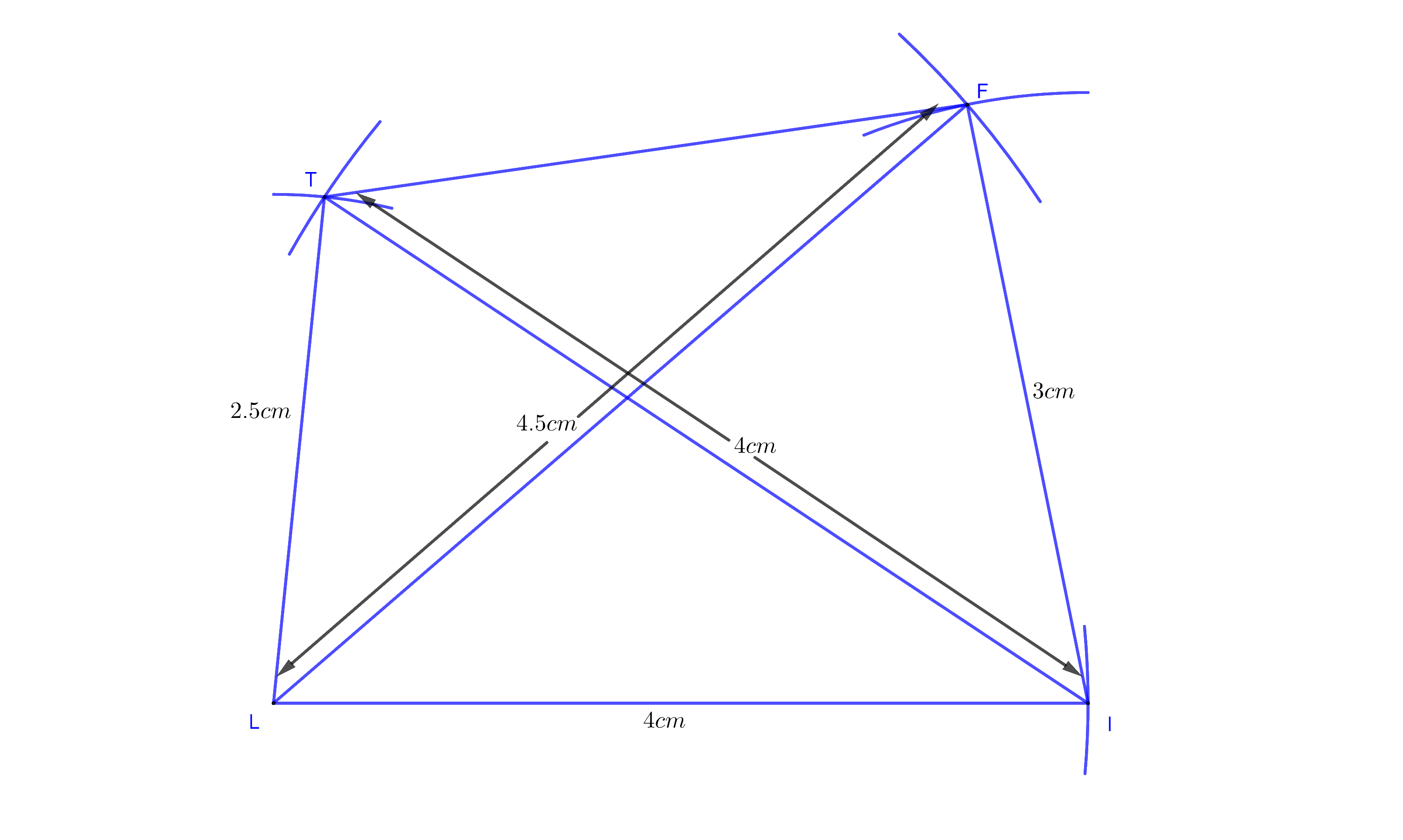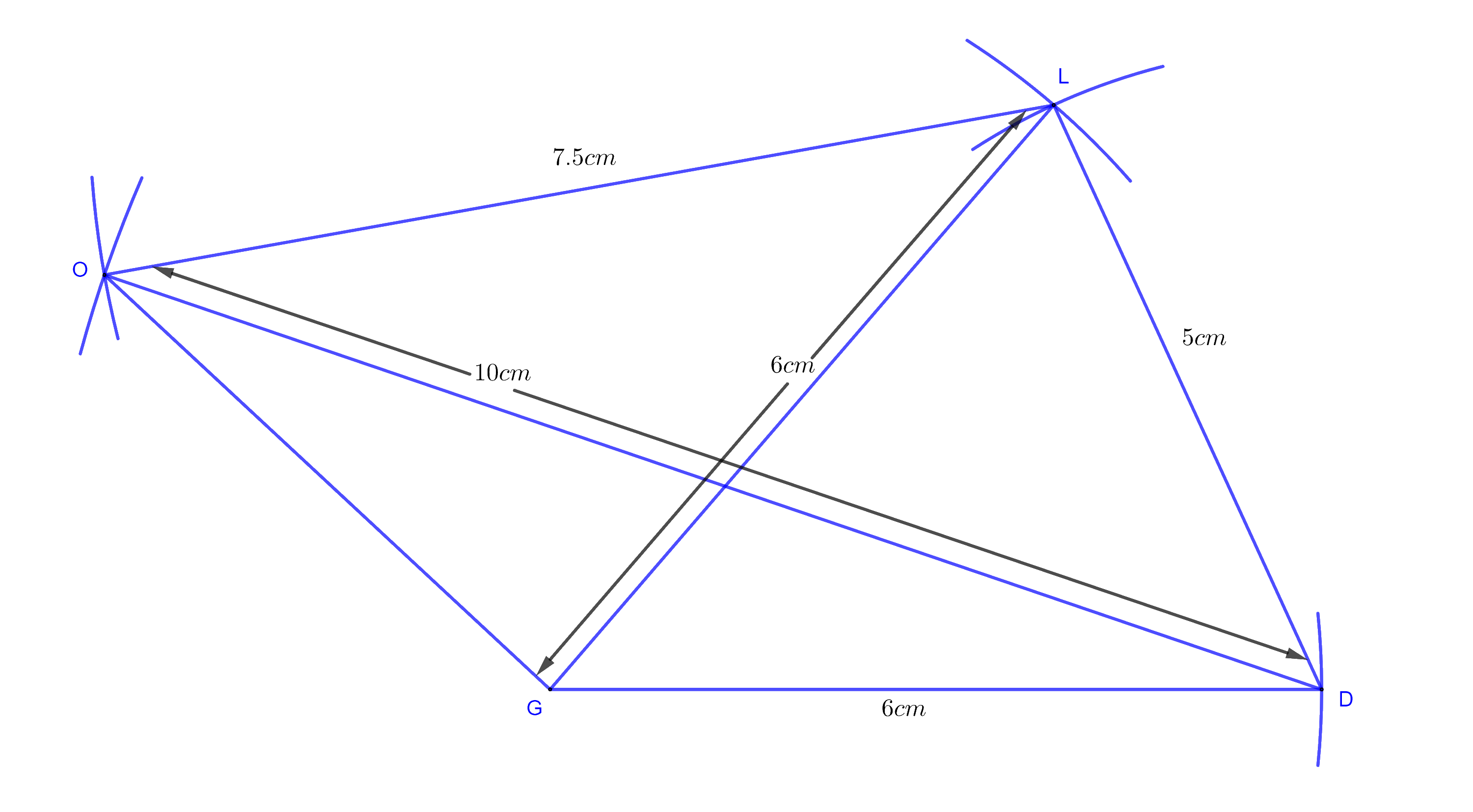# Excercise 4.2 Practical Geometry- NCERT Solutions Class 8

## Chapter 4 Ex.4.2 Question 1

Construct the following quadrilaterals.

(i) Quadrilateral $$LIFT$$

$${LI } =4\,\rm {cm}$$

$$IF =3\, \rm{cm}$$

$$TL=2.5\,\rm{cm}$$

$$LF=4.5\,\rm{cm}$$

$$IT =4\, \rm{cm}$$

(ii) Quadrilateral $$GOLD$$

$$OL = 7.5 \,\rm{cm}$$

$$GL = 6\,\rm{cm}$$

$$GD = 6\,\rm{cm}$$

$$LD = 5\,\rm{cm}$$

$$OD = 10 \rm \,cm$$

(iii) Rhombus $$BEND$$

$$BN = 5.6\,\rm{cm}$$

$$DE = 6.5\,\rm{cm}$$

### Solution

What is the known?

Measurements of a Quadrilateral.

What is unknown?

Construction of a Quadrilateral

Reasoning:

As you are aware, we need five measurements to draw a quadrilateral

Steps:

Let us draw a rough diagram with the given measurements to find out whether it is possible to construct a quadrilateral

Based on the given information it is easy to find out that the five given measurements are of $$3$$ sides and two diagonals. When measurements of $$3$$ sides and two diagonals of a quadrilateral are given, we can construct a quadrilateral The construction can be done in two parts. First draw $$\Delta {{LIF}}$$ and then draw $$\Delta {{LIT}}$$ and then join the other side.Let us see whether it is possible

In $$\Delta {{LIF,}}\; 4 + 3 > 4.5$$ and  $$4 - 3 < 4.5$$

$$4.5 + 3 > 4$$ and $$4.5 - 3 < 4$$

$$4.5 + 4 > 3$$ and $$4.5 - 4 < 3$$

In$$\Delta LIT,\;4 + 4 > 2.5$$  and $$4 - 4 < 2.5$$

$$4 + 2.5 > 4$$ and $$4 - 2.5 < 4$$

$$4 + 2.5 > 4$$ and $$4 - 2.5 < 4$$

In both cases, it is possible to form triangle.

Let us construct the quadrilateral

Step 1: Construct Line $$LI=4 \,\rm{cm.}$$ With $$L$$ as center and $$4.5\,\rm{ cm}$$ as radius draw an arc. With $$I$$ as center and $$3\,\rm{cm}$$ as radius draw an arc cutting the former one. The intersection point is $$F.$$ Join $$IF$$ and $$LF.$$

Step 2: With $$L$$ as center and radius $$2.5\,\rm{cm}$$ draw an arc. With $$I$$ as center and $$4\,\rm{cm}$$ as radius draw an arc cutting the former one at $$T.$$ Join $$LT$$ and $$IT.$$

Step 3: Join $$FT.$$

Step 4: $$LIFT$$ is the required quadrilateral

Related Problems

(ii) Quadrilateral $$GOLD$$\begin{align}OL&=7.5\,\rm{cm}\\GL&=6\,\rm{cm}\\GD&=6\,\rm{cm}\end{align}

\begin{align}LD&=5\,\rm{cm}\\OD&=10\,\rm{cm}\end{align}

(iii) Rhombus $$BEND$$\begin{align}BN&=5.6\,\rm{cm} \\DE&=6.5\,\rm{cm}\end{align}

Instant doubt clearing with Cuemath Advanced Math Program